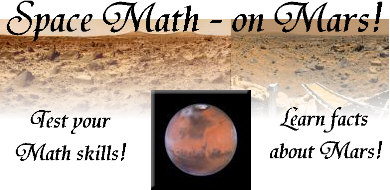NASA Project NOVA Activity Developer:  Donna Roberts

Use the interactive mathematics questions below to test your math skills while discovering
information about Mars and Mars exploration.
These questions are designed to test mathematical skills for grades 7 - 12.
Photographs are courtesy of NASA.

1.  The average diameter of Mars is 6,780 km.  This is approximately half the diameter of the Earth and twice the diameter of the Moon.

 a.  What is the ratio of the diameter of the Moon to the diameter of Mars? Answer The ratio is  1:2
 b.  What is the ratio of the diameter of the Mars to the diameter of the Earth? Answer The ratio is  1:2
 c.  What is the ratio of the diameter of the Moon to the diameter of the Earth? Answer The ratio is  1:4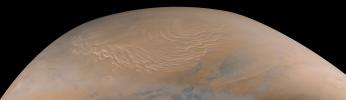The Martian North Polar Cap

 2.  In April of 1999, a cyclonic storm system near the north polar region of Mars was detected.   The storm measured 1100 by 900 miles in dimension.  What is the surface area of Mars covered by this storm, in square miles?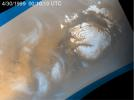Martian Storm Choose: 99,000  9.9x105  9.9x106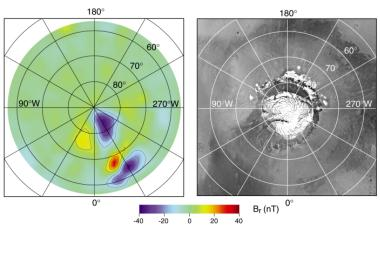3.  The map above shows the north polar magnetic anomalies of Mars.  What is the approximate latitude and longitude setting, in degrees, for the area on the map shaded in red? Choose: 30W by 68N 340W by 72N  340W by 68N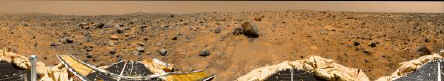4.  Because Mars is smaller and less dense than Earth, it has much less gravity.  A person that weighs 150 lbs on Earth will weigh 56.60378 lbs on Mars.  Based on this data, what is the approximate ratio of gravity on Mars to gravity on Earth? Choose: 1:3 2:3  3:1

5.  The surface temperature on Mars varies from -199 degrees Fahrenheit to 80 degrees Fahrenheit.  Using this data, what is the approximate average temperature on Mars?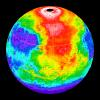Martian temperatures measured by a  thermal emissions spectrometer. Choose: +20.5 degrees  -59.5 degrees -139.5 degrees

6.  Mars revolves around the sun once every 687 Earth days.  Each year NASA scientists working on Earth are expected to present annual reports.  When the colonization of Mars occurs, scientists working on Mars will also be expected to present annual reports based on the Martian year.  Over a span of  n  Earth years, how many annual reports will Martian scientists present?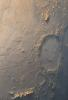"Happy Face" crater Choose: 1.88n reports 0.53n reports n reports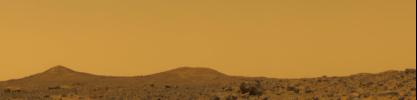7.  The Mars canyon system of Valles Marineris is the largest and deepest known in the solar system.  It extends more than 2,500 miles and reaches depths of 3 to 6 miles.  The average depth of the canyon was determined by averaging the depths taken at 150 locations along the canyon.  The cross-sectional area of the canyon, based upon a length of 2500 miles and the average depth, is 12,000 square miles.  What is the average depth of the canyon?

 Choose: 4 miles 4.5 miles 4.8 miles

8.  The highest point on Mars is Olympus Mons, a huge volcano.  The distance from the base of the volcano to the top of the crater is 18 miles.  The crater measures 370 miles across.  If a Martian hiker decides to climb this volcano on the north side and descend on the south side, how many miles will the hiker travel, to the nearest tenth of a mile?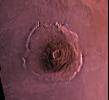Olympus Mons Choose: 36 miles 581.2 miles 617.2 miles 1198.4 miles

9.  Mars has two moons, Phobos and Deimos.  The distance from Mars to Phobos is 9378 km and to Deimos is 23,459 km.  The diameter of Phobos is 22.2 km and of Deimos is 12.6 km.  A radio signal is to be sent from the surface of Mars to the surface of Phobos and then to the surface of Deimos.  What is the shortest distance, in kilometers, that the radio signal could travel to accomplish this task?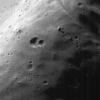Face of Phobos Choose: 14081 km 14115.8 km 23459 km 23481.2 km

10.  Mars is about 1.5 times as far from the Sun as the Earth is.  Approximately how far from the Sun is Mars?Choose: 9 x 107 miles 9 x 108 miles 1.35 x 107 miles 1.35 x 108 miles